百度有2个地理位置相距多少公里的API吗？

kacc850 发布于 2014/08/14 09:01

【推荐阅读】滴滴出行的数据架构和信息流处理思路解析->>>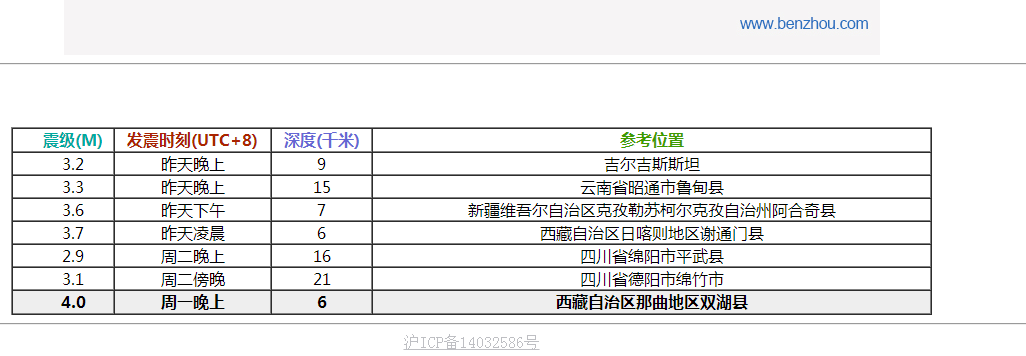要做个安全范围

以下是问题补充：

@kacc850：1：抓取地震经纬度 2：获取用户IP所在城市 3：把城市转化为经纬度 4：计算2个经纬度之差，算出距离和相对方向 5：利用震级+地震深度计算多少范围内是安全范围 6：与4结果进行对比 7：返回给网友所在地区距离地震是否是安全范围还是危险范围。 (2014/08/14 09:40)

0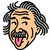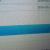0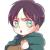0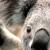0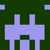0
0引用来自“purely”的评论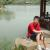0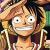BMap 类有一个方法 getDistance()，可以获取两个坐标点之间的距离，建议你看下。给段代码：

// 百度地图API功能
var map = new BMap.Map("allmap");
var point = new BMap.Point(120.219375,30.259244);
map.centerAndZoom(point,12);
map.pixelToPoint();
// 创建地址解析器实例
var myGeo = new BMap.Geocoder(), start = null, end = null;
// 将地址解析结果显示在地图上,并调整地图视野，获取 start 点
myGeo.getPoint("杭州市滨江区", function(point){
if (point) {
// start 点坐标
start = point;
map.centerAndZoom(point, 12);
myGeo.getPoint("杭州市西湖区", function(point){
if (point) {
// end 点坐标
end = point;
// 获取 start 和 end 直接的距离，单位：米
console.log(map.getDistance(start, end));
}
}, "杭州市");
}
}, "杭州市");

0
j

public class LocationUtils {

private final static double EARTH_RADIUS = 6378.137;

/**
* 获取两地距离
*
* @param lat1
*            A地纬度
* @param lng1
*            A地经度
* @param lat2
*            B地纬度
* @param lng2
*            B地经度
* @return
*/
public static double getDistance(double lat1, double lng1, double lat2,
double lng2) {
double s = 2 * Math.asin(Math.sqrt(Math.pow(Math.sin(a / 2), 2)
* Math.pow(Math.sin(b / 2), 2)));
s = s * EARTH_RADIUS;
s = s * 1000;
return s;
}

/**
* 获取两地距离
*
* @param lat1
*            A地纬度
* @param lng1
*            A地经度
* @param lat2
*            B地纬度
* @param lng2
*            B地经度
* @return
*/
public static double getKilometreDistance(double lat1, double lng1,
double lat2, double lng2) {
double s = 2 * Math.asin(Math.sqrt(Math.pow(Math.sin(a / 2), 2)
* Math.pow(Math.sin(b / 2), 2)));
s = s * EARTH_RADIUS;
s = Math.round(s * 10000) / 10000;
return s;
}

public static void main(String[] args) {
System.out.println(getDistance(23.134507, 113.372508, 23.13073,
113.402195));
}

private static double rad(double d) {
return d * Math.PI / 180.0;
}

}